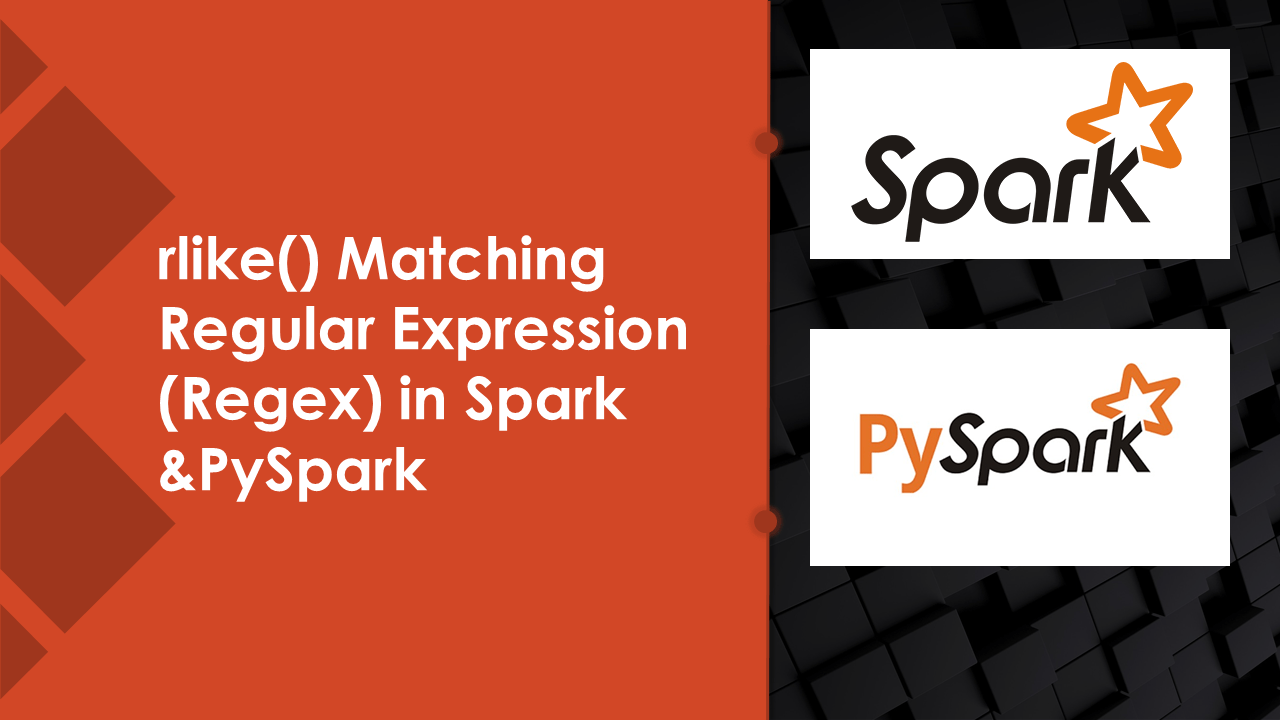# Spark rlike() Working with Regex Matching Examples

Similar to SQL `regexp_like()` function Spark & PySpark also supports Regex (Regular expression matching) by using `rlike()` function, This function is available in org.apache.spark.sql.Column class. Use regex expression with rlike() to filter rows by checking case insensitive (ignore case) and to filter rows that have only numeric/digits and more examples.

Key points:

• rlike() is a function of org.apache.spark.sql.Column class.
• rlike() is similar to like() but with regex (regular expression) support.
• It can be used on Spark SQL Query expression as well.
• It is similar to regexp_like() function of SQL.

## 1. rlike() Syntax

Following is a syntax of `rlike()` function, It takes a literal regex expression string as a parameter and returns a boolean column based on a regex match.

``````
// Syntax
def rlike(literal : _root_.scala.Predef.String) : org.apache.spark.sql.Column
``````

## 2. rlike() Usage

rlike() function can be used to derive a new Spark/PySpark DataFrame column from an existing column, filter data by matching it with regular expressions, use with conditions, and many more.

``````
// Usage
import org.apache.spark.sql.functions.col
col("alphanumeric").rlike("^[0-9]*\$")
df("alphanumeric").rlike("^[0-9]*\$")
``````

## 3. Spark rlike() Examples

Following are different examples of using rlike() function with Spark (with Scala) & PySpark (Spark with Python) and SQL. For PySpark use `from pyspark.sql.functions import col` to use col() function.

### 3.1 Filter Rows that Contain Only Numbers

Using with DataFrame API

``````
// Filter DataFrame rows that has only digits of 'alphanumeric' column
import org.apache.spark.sql.functions.col
df.filter(col("alphanumeric")
.rlike("^[0-9]*\$")
).show()
``````

### 3.2 Filter Rows by Case Insensitive

Below is an example of a regular expression to filter the rows by comparing case insensitive (filter rows that contain `rose` string in a column `name`).

``````
// Filter rows by cheking value contains in anohter column by ignoring case
import org.apache.spark.sql.functions.col
df.filter(col("name").rlike("(?i)^*rose\$")).show()
``````

## 4. PySpark SQL rlike() Function Example

Let’s see an example of using rlike() to evaluate a regular expression, In the below examples, I use rlike() function to filter the PySpark DataFrame rows by matching on regular expression (regex) by ignoring case and filter column that has only numbers.

rlike() evaluates the regex on Column value and returns a Column of type Boolean.

rlike() is a function on Column type, for more examples refer to PySpark Column Type & it’s Functions

``````
# PySpark Example
from pyspark.sql.functions import col

# Filter DataFrame rows that has only digits of 'alphanumeric' column
df.filter(col("alphanumeric").rlike("^[0-9]*\$"))
.show()

# Filter rows by cheking value contains in anohter column by ignoring case
df.filter(col("name").rlike("(?i)^*rose\$"))
.show()
``````

## 5. Spark SQL rlike() Function

Similar to SQL regexp_like(), Spark SQL have rlike() that takes regular expression (regex) as input and matches the input column value with the regular expression.

``````
// Filter rows that only digits of 'alphanumeric' column
df.createOrReplaceTempView("DATA")
spark.sql("select * from DATA where rlike(alphanumeric,'^[0-9]*\$')").show()
``````

## Conclusion

In this Spark, PySpark article, I have covered examples of how to rlike() regex expression to filter DataFrame rows by comparing case insensitive string contains in another string & filtering rows that have only numeric values e.t.c

Happy Learning !!

## Related Articles

### Naveen (NNK)

Naveen (NNK) is a Data Engineer with 20+ years of experience in transforming data into actionable insights. Over the years, He has honed his expertise in designing, implementing, and maintaining data pipelines with frameworks like Apache Spark, PySpark, Pandas, R, Hive and Machine Learning. Naveen journey in the field of data engineering has been a continuous learning, innovation, and a strong commitment to data integrity. In this blog, he shares his experiences with the data as he come across. Follow Naveen @ @ LinkedIn